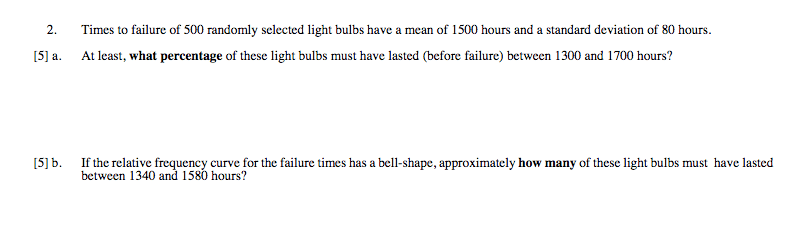# Calculations using Standard Deviation and Mean

• Enharmonics
In summary, the homework statement is:Homework Equations:-The percentage of observations that are within k standard deviations of the mean is at least100(1 - (1/k2))%-Chebyshev's Theorem is applicable to ANY data set, whether skewed or symmetrical.-For a symmetrical data set, approximately68% of the data is within 1 standard deviation from the mean95% of the data is within 2 standard deviations from the mean99.7% of the data is within 3 standard deviations from the mean.f

## Homework Statement## Homework Equations

Chebyshev's Theorem: The percentage of observations that are within k standard deviations of the mean is at least

100(1 - (1/k2))%

Chebyshev's Theorem is applicable to ANY data set, whether skewed or symmetrical.

Empirical Rule: For a symmetrical data set, approximately

• 68% of the data is within 1 standard deviation from the mean
• 95% of the data is within 2 standard deviations from the mean
• 99.7% of the data is within 3 standard deviations from the mean

## The Attempt at a Solution

[/B]
I'm a little confused as to how to solve these problems. Based on the wording, I think I'm supposed to be using Chebyshev's Theorem and the Empirical Rule, but I'm not sure.

I solved (a) using Chebyshev's Theorem, since at that point in the assignment we haven't been told whether the data is symmetric (has a bell shape) or not:

We've got

mean = 1500
standard deviation = 80

Since the problem is asking for the light bulbs that lasted between 1300 and 1700 hours until failure - that is, the interval (1300, 1700) - I note that for k = 3 standard deviations from the mean, we have an interval of hours of operation until failure of

(mean - 3 (standard deviation), mean + 3 (standard deviation)) = (1500 - 3(80) , 1500 + 3(80)) = (1260, 1740)

which would indicate that the percentage of the light bulbs that lasted between the specified interval is given by

100(1 - 1/(3)2)% = 100(1- (1/9))% ≈ 89%

This is where I'm a little confused. The problem is asking for the number of light bulbs whose hours of operation fall within the interval (1300, 1700), but the interval given by adding/subtracting 3 standard deviations is (1260, 1740), which is a little too far; by contrast, the interval given by using k = 2 isn't far enough.

Now, I have noticed that the interval (1300, 1700) ⊆ (1260, 1740) since every point in the former is a member of the latter, but does that make it okay to apply Chebyshev's Rule here?

I have a similar concern about (b). Since the data set is bell-shaped and thus roughly symmetrical, I used the Empirical Rule.

The interval given by the problem is (1340, 1580).

Since the interval calculated by using 2 standard deviations from the mean is

(1500 - 2(80) , 1500 + 2(80)) = (1340, 1660)

and (1340, 1580) ⊆ (1340, 1660) by the same reasoning used earlier, I conclude that based on the Empirical Rule, at least 95.4% of values must fall within the specified range, which would yield

500 * (0.954) = 477 lightbulbs

lasted between 1340 and 1580 hours. Is this correct, or am I misusing these rules? I've seen some classmates doing calculations that involved p-values and z-scores, am I supposed to be using those instead?

#### Attachments

Empirical Rule: For a symmetrical data set, approximately
That is only true for Gaussian distributions. You can't use this in (a).

Symmetry and bell shape are not the same. There are many symmetric distributions that don't have a bell shape.
I solved (a) using Chebyshev's Theorem
Good.
This is where I'm a little confused. The problem is asking for the number of light bulbs whose hours of operation fall within the interval (1300, 1700), but the interval given by adding/subtracting 3 standard deviations is (1260, 1740), which is a little too far; by contrast, the interval given by using k = 2 isn't far enough.
Chebyshev's Theorem works with every (non-negative real) value k, you don't have to use integers. And you can't use integer k values here.
I have a similar concern about (b). Since the data set is bell-shaped and thus roughly symmetrical, I used the Empirical Rule.
It is not roughly symmetrical, it is exactly symmetrical and follows a Gaussian distribution (I guess that's what you call "bell shape"). Same as above: You are not limited to integers.

•Enharmonics
mean = 1500
standard deviation = 80

Since the problem is asking for the light bulbs that lasted between 1300 and 1700 hours until failure - that is, the interval (1300, 1700)

If you are using tables of a normal distribution, you can find the probability that a sample lies between non-integer multiples of a standard deviation from the mean. You should be able to use a table or a calculator to find the probability that a lifetime is within ##\pm 200/80## ths of standard deviation from the mean.

Perhaps you text materials have covered "z-scores". The z-score of a value X drawn from a normal distribution of mean ##\mu## and standard devation ##\sigma## is defined to be ##z_X = \frac{(x - \mu)}{\sigma}##. The z-score measures how many standard deviations ##X## is from the mean.

Tables of the standard normal distribution will let you find the probability that sample is between two z-scores - such as -1.73 to 0.82. You can't read such an answer from the table in one step. You have to do some reasoning and simple arthmetic.

•Enharmonics